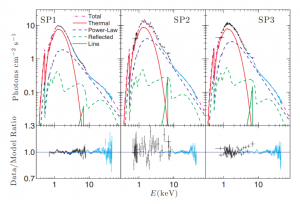# 2019年USAAAO决赛第11题

## 英文题目

11. (25 points)

Cygnus X-1/HDE 226868 is a binary system consisting of a black hole Cygnus X-1 and blue supergiant HDE 226868. The mass of HDE 226868 is 30M@ and the period of the binary system is 5.6 days. Radial velocity data reveals that the orbital velocity of HDE 226868 is 116.68 km/s at apoapse and 123.03 km/s at periapse.

(a) (5 points) Determine the eccentricity of the orbit of HDE 226868.

(b) (5 points) Determine the length of the semimajor axis of the orbit of HDE 226868.

(c) (5 points) Determine the mass of Cygnus X-1, to at least 3 significant figures. The peak blackbody temperature of an accretion disk occurs at a distance of $$r_{peak}$$ and a temperature of $$T_{peak}$$. One can determine the peak blackbody temperature by assuming that it corresponds to the peak in the x-ray spectrum. Due to relativistic effects, the actual peak blackbody temperature $$T_{peak}$$ is related to the peak color temperature Tcolor derived from observed spectral data by $$Tcolor = f_{GR}f_{col}T_{peak}$$, where $$f_{GR} \approx 0.510$$ and $$f_{col} \approx 1.7$$. Three x-ray spectra of Cygnus X-1 are shown in Figure 4.Figure 4: Three x-ray spectra from Cygnus X-1. From Gou et al. (2011).

(d) (4 points) Using spectrum SP2, determine the peak blackbody temperature $$T_{peak}$$ of the accretion disk around Cygnus X-1.

The total luminosity of the blackbody component of the accretion disk can be estimated by $$L_{disk} \approx 4πσr^2_{pea}kT^4_{peak}$$ (Makishima et al. 1986). The radius $$r_last$$ of the innermost edge of the accretion disk is related to the radius $$r_{peak}$$ of the peak blackbody temperature by $$r_{peak} = ηr_{last}$$, where $$η \approx 0.63$$. In 1996, the blackbody luminosity of the accretion disk around Cygnus X-1 was estimated to be $$2.2 \times 10^{37} ergs/s$$.

(e) (4 points) Determine the radius $$r_{last}$$ of the innermost edge of the accretion disk around Cygnus X-1. Assume that the innermost edge of the accretion disk is located at the innermost stable circular orbit (ISCO), whose radius $$r_{isco}$$ is a function of the spin of the black hole. The relationship between $$r_{isco}$$ and $$a_*$$, the spin parameter of the black hole, can be estimated by: $$r_{isco} =\dfrac{GM^2}{c^2}(\sqrt{8.354 [(2-a_*^2)^2-1]}+1)$$

(f) (2 points) Determine the spin parameter $$a_*$$ of Cygnus X-1.